Courses

# Chemistry Test 3 - Atomic Structure, Mole Concept, Chemical Bonding, Periodic Properties, States Of Matter, Equilibrium, Hydrocarbons, General Organic Compounds, Alkyl Halide

## 25 Questions MCQ Test Mock Test Series for JEE Main & Advanced 2022 | Chemistry Test 3 - Atomic Structure, Mole Concept, Chemical Bonding, Periodic Properties, States Of Matter, Equilibrium, Hydrocarbons, General Organic Compounds, Alkyl Halide

Description
This mock test of Chemistry Test 3 - Atomic Structure, Mole Concept, Chemical Bonding, Periodic Properties, States Of Matter, Equilibrium, Hydrocarbons, General Organic Compounds, Alkyl Halide for JEE helps you for every JEE entrance exam. This contains 25 Multiple Choice Questions for JEE Chemistry Test 3 - Atomic Structure, Mole Concept, Chemical Bonding, Periodic Properties, States Of Matter, Equilibrium, Hydrocarbons, General Organic Compounds, Alkyl Halide (mcq) to study with solutions a complete question bank. The solved questions answers in this Chemistry Test 3 - Atomic Structure, Mole Concept, Chemical Bonding, Periodic Properties, States Of Matter, Equilibrium, Hydrocarbons, General Organic Compounds, Alkyl Halide quiz give you a good mix of easy questions and tough questions. JEE students definitely take this Chemistry Test 3 - Atomic Structure, Mole Concept, Chemical Bonding, Periodic Properties, States Of Matter, Equilibrium, Hydrocarbons, General Organic Compounds, Alkyl Halide exercise for a better result in the exam. You can find other Chemistry Test 3 - Atomic Structure, Mole Concept, Chemical Bonding, Periodic Properties, States Of Matter, Equilibrium, Hydrocarbons, General Organic Compounds, Alkyl Halide extra questions, long questions & short questions for JEE on EduRev as well by searching above.
QUESTION: 1

### IUPAC name of compound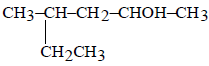is

Solution: The functional group is OH and it should get low locant and longest chain should be selected for naming so methane becomes substituient and get locant number 4
QUESTION: 2

Solution:
QUESTION: 3

### The nature of inter-molecular forces among benzene molecules is

Solution: Intermolecular Forces - Benzene. There are three different types of intermolecular forces, dipole interaction, hydrogen bonding, and dispersion forces. The only one that occurs with Benzene is dispersion forces because it is a non-polar molecule.
QUESTION: 4
The geometrical isomerism is shown
Solution:
QUESTION: 5

The addition of HCN to a carbonyl compound is an example of

Solution: As carbonyl contains pi bond which broke and later get added therefore it is a ncleophilic addtion reaction.
QUESTION: 6
Acetaldehyde is the rearrangement product of
Solution:
QUESTION: 7
In the presence of platinum catalyst, hydrocarbon A adds hydrogen to form n-hexane. When hydrogen bromide is added to A instead of hydrogen, only a single bromo compound is formed. Which of the following is A ?
Solution:
QUESTION: 8
Which of the following will convert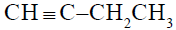to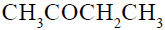?
Solution:
QUESTION: 9

1,3-Butadiene when treated with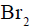gives

Solution:
QUESTION: 10
The alkene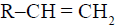reacts readily with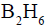and formed the product B which on oxidation with alkaline hydrogen peroxides produces
Solution:
QUESTION: 11
The ortho-para directing group among the following is
Solution:
QUESTION: 12
Amongst the following, the compound that can be most readily sulphonated is
Solution:
−CH3 group that has +1 effect and due to its presence, toluene has highest electron density in the benzene ring.
For this reason Toluene can be easily sulphonated.
QUESTION: 13
Chloroform on warming with Ag powder gives
Solution:
QUESTION: 14

Ethyl alcohol gives ethyl chloride with the help of

Solution:

With SOCl2, alcohols give Haloalkanes the best way and also from NaCl we obtain only Cl- which is weak nucleophile and cannot replace OH- which is a stronger nucleophile.

QUESTION: 15
Which of the following compound will make precipitate most readily with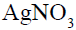?
Solution:
QUESTION: 16
Which of the following is responsible for depletion of the ozone layer in the upper strata of the atmosphere?
Solution:
QUESTION: 17
7.5 grams of a gas occupy 5.6 litres of volume at STP. The gas is
Solution:
QUESTION: 18
The number of water molecules present in a drop of water (volume 0.0018 ml) at room temperature is
Solution: The density of water is 1g/cm3 Volume of a drop of water =0.0018ml Mass of water drop=0.0018g Moles of water=0.0001 No of water molecules in 1 drop =0.0001X6.022X10^23 =6.022X10^19 water molecules.
QUESTION: 19
The correct representation of Charle’s law is given by
Solution:
QUESTION: 20
The structure of TlCl is similar to CsCl. What would be the radius ratio in TlCl ?
Solution:
*Answer can only contain numeric values
QUESTION: 21

Sulphur trioxide is prepared by the following two reactions:-
S8(s) + 8 O2(g) —→ 8 SO2(g)
2SO2(g) + O2(g) —→ 2 SO3(g)
How many grams of SO3 are produced from 1 mole S8?

Solution:

from the given reaction, it is clear that 1 mol Swill give 8 moles of SO3.

*Answer can only contain numeric values
QUESTION: 22

How many elements are more electropositive than Cl?
Be, F, O, S, P, Au, H, Na

Solution:

6.0
F and O are more electronegative than Cl.

*Answer can only contain numeric values
QUESTION: 23

How many elements have more ionisation energy as compared to their next higher atomic number element?
Na, Mg, Al, Si, P, S, Cl, Ar

Solution:

2.0 Order of ionization energy
Na < M g > Al < Si < P > S < Cl < Ar

*Answer can only contain numeric values
QUESTION: 24

How many of the following compounds are more reactive than benzene towards electrophilic substitution.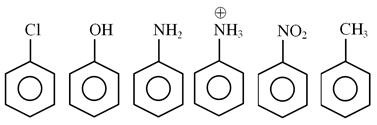Solution: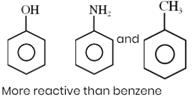*Answer can only contain numeric values
QUESTION: 25

Number of d-orbitals used in the hybridisation of ICl3 is = x and number of lone pair at central atom = y find x + y = ?

Solution: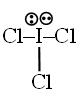sp3d    [x = 1; y = 2]
lp = 2
x + y = 1 + 2 = 3